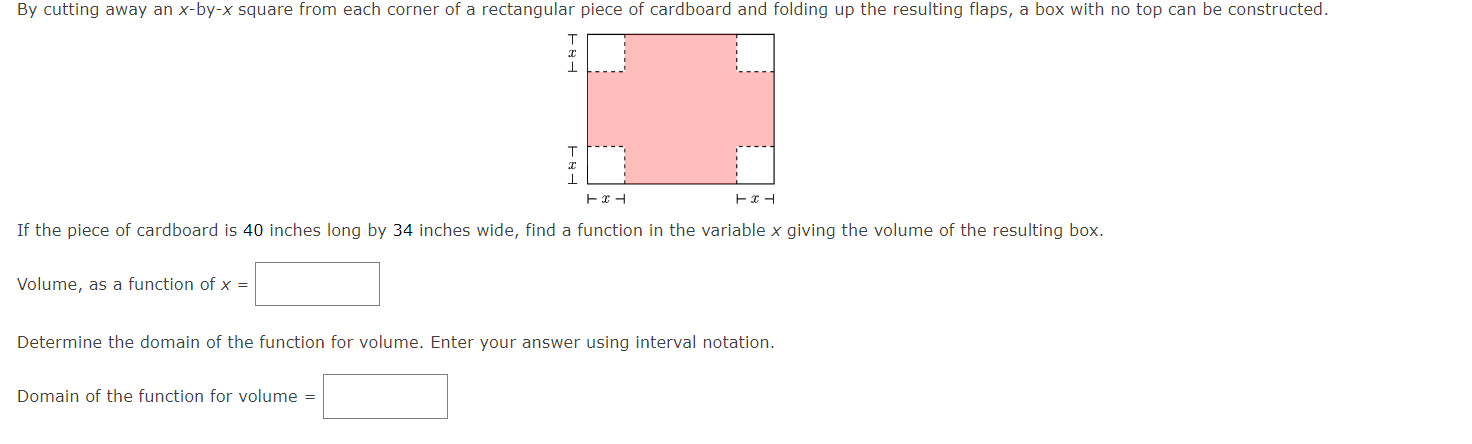Home / Expert Answers / Calculus / by-cutting-away-an-x-by-x-square-from-each-corner-of-a-rectangular-piece-of-cardboard-pa708

# (Solved): By cutting away an \( x \)-by- \( x \) square from each corner of a rectangular piece of cardboard ...By cutting away an \( x \)-by- \( x \) square from each corner of a rectangular piece of cardboard and folding up the resulting flaps, a box with no top can be constructed. If the piece of cardboard is 40 inches long by 34 inches wide, find a function in the variable \( x \) giving the volume of the resulting box. Volume, as a function of \( x= \) Determine the domain of the function for volume. Enter your answer using interval notation. Domain of the function for volume \( = \)

We have an Answer from Expert